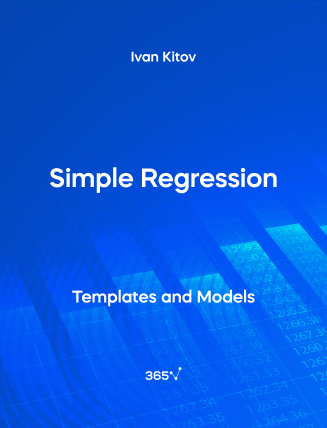## Simple Regression – Excel Template###### Ivan Kitov

The Simple Regression quantifies the relationship between a variable, known as dependent variable, and one explanatory variables, called independent variable.

Regression analysis can help us understand the impact of multiple factors on the outcome of an event. For example, the relationship between house price and house size.

Some other related topics you might be interested to explore are the Capital Asset Pricing Model, Regression Analysis (two variables), and Security Market Line.

This is an open-access Excel template in XLSX format that will be useful for anyone who wants to work as a Statistician, Financial Analyst, Data Analyst, or Portfolio Manager.